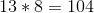ISEE Middle Level Math : How to find the square root

Example Questions

Example Question #91 : How To Find The Square Root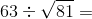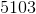Explanation:

First, find the square root: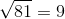Then, solve: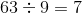Example Question #91 : Squares / Square Roots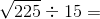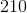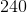Explanation:

First, find the square root: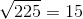Then, solve: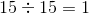Example Question #92 : Squares / Square Roots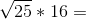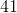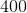Explanation:

First, find the square root: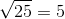Then, solve: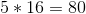Example Question #93 : Squares / Square Roots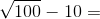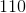Explanation:

First, find the square root: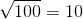Then, solve: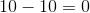Example Question #94 : Squares / Square Roots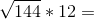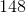Explanation:

First, find the square root: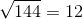Then, solve: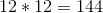Example Question #95 : Squares / Square Roots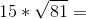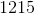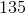Explanation:

First, find the square root:Then, solve: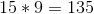Example Question #96 : Squares / Square Roots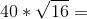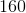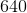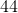Explanation:

First, find the square root: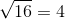Then, solve: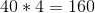Example Question #98 : How To Find The Square Root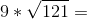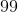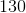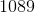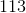Explanation:

First, find the square root: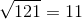Then, solve: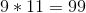Example Question #99 : How To Find The Square Root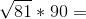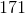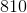Explanation:

First, find the square root:Then, solve: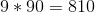Example Question #100 : How To Find The Square Root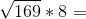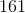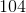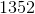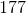Explanation:

First, find the square root: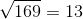Then, solve: